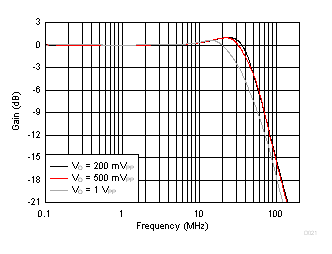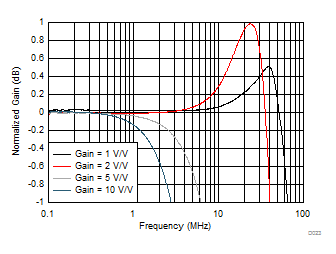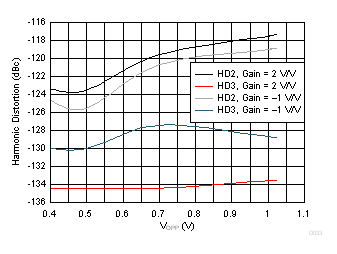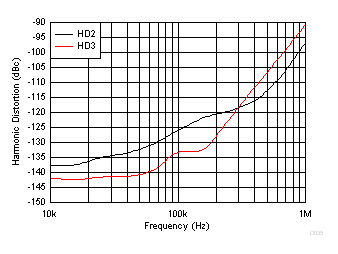ZHCSGP3D September   2017  – December 2018

PRODUCTION DATA.

1. 特性
2. 应用
3. 说明
4. 修订历史记录
5. Pin Configuration and Functions
6. Specifications
7. Detailed Description
1. 7.1 Overview
2. 7.2 Functional Block Diagrams
3. 7.3 Feature Description
4. 7.4 Device Functional Modes
8. Application and Implementation
1. 8.1 Application Information
2. 8.2 Typical Applications
1. 8.2.1 Active Filters
2. 8.2.2 Implementing a 2:1 Active Multiplexer
3. 8.2.3 1-Bit PGA Operation
9. Power Supply Recommendations
10. 10Layout
11. 11器件和文档支持
1. 11.1 文档支持
2. 11.2 相关链接
3. 11.3 接收文档更新通知
4. 11.4 社区资源
5. 11.5 商标
6. 11.6 静电放电警告
7. 11.7 术语表
12. 12机械、封装和可订购信息

• DCK|5
• DBV|6
• DCK|5

### 6.9 Typical Characteristics: VS = 3.0 V

at VS+ = 3.0 V, VS– = 0 V, VOUT = 1 VPP, RF = 0 Ω, RL = 2 kΩ, G = 1 V/V, input and output referenced to mid-supply, and TA ≈ 25°C (unless otherwise noted)See Figure 74 and Table 2, VOUT = 20 mVPP, RLOAD = 2 kΩ
Figure 19. Noninverting Small-Signal Response vs GainSee Figure 74, gain = 2 V/V
Figure 21. Noninverting Large-Signal Bandwidth vs VOPPSee Figure 74 and Table 2, VOUT = 200 mVPP, RLOAD = 2 kΩ
Figure 23. Noninverting Response Flatness vs GainSee Figure 74 and Table 2, gain = 2 V/V, input edge rate set to stay below slew limiting
Figure 25. Noninverting Step Response vs VOPPSee Figure 74 and Table 2
Figure 27. Simulated Noninverting Settling TimeSee Figure 74 and Table 2, gain = 2 V/V
Figure 29. Noninverting Overdrive RecoverySee Figure 74, Figure 75, Table 2, and Table 3, VOUT = 1 VPP, RLOAD = 2 kΩ
Figure 31. Harmonic Distortion vs FrequencySee Figure 74, Figure 75, Table 2, and Table 3, RLOAD = 2 kΩ, f = 100 kHz
Figure 33. Harmonic Distortion vs Output SwingSee Figure 87, gain = 1 V/V, VOUT = 1 VPP, RLOAD = 2 kΩ
Figure 35. Harmonic Distortion as Active MuxSee Figure 75 and Table 3, VOUT = 20 mVPP, RLOAD = 2 kΩ
Figure 20. Inverting Small-Signal Response vs GainSee Figure 75, gain = –1 V/V
Figure 22. Inverting Large-Signal Bandwidth vs VOPPSee Figure 75 and Table 3, VOUT = 200 mVPP, RLOAD = 2 kΩ
Figure 24. Inverting Response Flatness vs GainSee Figure 75 and Table 3, gain = –1 V/V, input edge rate set to stay below slew limiting
Figure 26. Inverting Step Response vs VOPPSee Figure 75 and Table 3
Figure 28. Simulated Inverting Settling TimeSee Figure 75 and Table 3, gain = –1 V/V
Figure 30. Inverting Overdrive RecoverySee Figure 74, Figure 75, Table 2, and Table 3, VOUT = 1 VPP, f = 100 kHz, RLOAD = 2 kΩ
Figure 32. Harmonic Distortion vs RLOADSee Figure 74, Figure 75, Table 2, and Table 3, RLOAD = 2 kΩ, f = 100 kHz, VOUT = 1 VPP
Figure 34. Harmonic Distortion vs Gain MagnitudeSee Figure 88, gain of 1 V/V and 2 V/V, VOUT = 1 VPP, RLOAD = 2 kΩ
Figure 36. Harmonic Distortion as 1-Bit PGA